##### Question

In: Statistics and Probability

# Construct a 90​% confidence interval to estimate the population mean using the accompanying data. What assumptions...

Construct a 90​% confidence interval to estimate the population mean using the accompanying data. What assumptions need to be made to construct this​ interval?

x=59 σ=15 n=17

What assumptions need to be made to construct this​ interval?

A. The population is skewed to one side.

B. The sample size is less than 30.

C. The population must be normally distributed.

D. The population mean will be in the confidence interval.

With 90​% ​confidence, when n=17​, the population mean is between the lower limit of and the upper limit of .

## Solutions

##### Expert Solution

Solution :

Given that,

Point estimate = sample mean =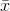= 59

Population standard deviation =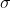= 15

Sample size = n = 17

At 90% confidence level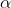= 1-0.90% =1-0.90 =0.10/2 =0.10/ 2= 0.05

Z/2 = Z0.05 = 1.645

Z/2 = 1.645
Margin of error = E = Z/2 * (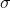/n)

= 1.645 * ( 15/ 17 )

= 5.98

At 90 % confidence interval estimate of the population mean is,- E <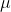<+ E

59 - 5.98 << 59+ 5.98

53.02 << 64.98

( 53.02,64.98 )

Answer D. The population mean will be in the confidence interval.

With 90​% ​confidence, when n=17​, the population mean is between the lower limit of =53.02 and the upper limit of =64.98.

## Related Solutions

##### Construct a 95?% confidence interval to estimate the population mean using the following data. What assumptions...
Construct a 95?% confidence interval to estimate the population mean using the following data. What assumptions need to be made to construct this? interval? x?=93 ?=15 n=17 With 95?% ?confidence, when n=17 the population mean is between the lower limit of __ and the upper limit of __
##### Construct a 99​% confidence interval to estimate the population mean using the data below. What assumptions...
Construct a 99​% confidence interval to estimate the population mean using the data below. What assumptions need to be made about this​ population? x overbar =32 s=9.1 n=28
##### Construct a 95% confidence interval to estimate the population mean using the following data. What assumptions...
Construct a 95% confidence interval to estimate the population mean using the following data. What assumptions need to be made to construct this​ interval? x(over bar x)= 83   s=19    n=16 What assumptions need to be made to construct this​ interval? A. The population is skewed to one side. B. The sample size is less than 30. C. The population mean will be in the confidence interval. D. The population must be normally distributed. With 95​% ​confidence, when n=16 the population...
##### Refer to the accompanying data set and construct a 90​% confidence interval estimate of the mean...
Refer to the accompanying data set and construct a 90​% confidence interval estimate of the mean pulse rate of adult​ females; then do the same for adult males. Compare the results. LOADING... Click the icon to view the pulse rates for adult females and adult males. Construct a 90​% confidence interval of the mean pulse rate for adult females. nothing bpmless thanmuless than nothing bpm ​(Round to one decimal place as​ needed.) Construct a 90​% confidence interval of the mean...
##### Refer to the accompanying data set and construct a 90​% confidence interval estimate of the mean...
Refer to the accompanying data set and construct a 90​% confidence interval estimate of the mean pulse rate of adult​ females; then do the same for adult males. Compare the results. Males   Females 87   77 74   92 51   56 60   65 54   54 61   83 51   78 77   85 49   89 62   55 74   34 59   68 66   81 76   79 80   79 63   66 64   66 96   80 45   58 85   61 72   82 62   85 73   72 71  ...
##### Refer to the accompanying data set and construct a 90​% confidence interval estimate of the mean...
Refer to the accompanying data set and construct a 90​% confidence interval estimate of the mean pulse rate of adult​ females; then do the same for adult males. Compare the results. Males Females 82       83 72       94 48       59 57       64 54       56 62       80 53       78 74       86 53       86 63       58 73       35 61       65 65       85 76       76 83       75 65       64 64       68 93       79 41       59 84       63 74       82 64       82 72       68 70      ...
##### Refer to the accompanying data set and construct a 90​% confidence interval estimate of the mean...
Refer to the accompanying data set and construct a 90​% confidence interval estimate of the mean pulse rate of adult​ females; then do the same for adult males. Compare the results. Pulse Rates (beats per minute) Males Females 82 83 72         94 48       59 57        64 54 56 62 80 53 78 74 86 53 86 63 58 73 35 61 65 65 85 76 76 83 75 65 64 64 68 93 79 41 59 84 63 74 82...
##### Refer to the accompanying data set and construct a 90​% confidence interval estimate of the mean...
Refer to the accompanying data set and construct a 90​% confidence interval estimate of the mean pulse rate of adult​ females; then do the same for adult males. Compare the results. Males Females 85 71 79 82 71 66 94 79 52 75 59 70 58 74 65 77 53 56 54 86 62 66 83 88 52 58 76 89 79 79 86 91 53 70 88 9 61 65 57 96 72 61 38 69 57 98 64...
##### Refer to the accompanying data set and construct a 90​% confidence interval estimate of the mean...
Refer to the accompanying data set and construct a 90​% confidence interval estimate of the mean pulse rate of adult​ females; then do the same for adult males. Compare the results. Males Females 83 79 74 96 51 56 61 67 53 55 59 83 53 78 78 84 52 90 63 58 69 37 61 64 67 86 76 76 80 78 65 64 68 66 97 76 45 62 86 66 75 83 61 80 70 72 73...
##### Construct a 95% confidence interval to estimate the population mean using the data below. What assumptions need to be made about this population?
Construct a 95% confidence interval to estimate the population mean using the data below. What assumptions need to be made about this population? x̄ = 33 s= 9.9 n=27 The 95% confidence interval for the population mean is from a lower limit of _______  to an upper limit of _______ .